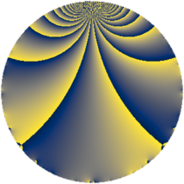# Properties

 Label 2001.2.eLevel $2001$ Weight $2$ Character orbit 2001.e Rep. character $\chi_{2001}(898,\cdot)$ Character field $\Q$ Dimension $112$ Sturm bound $480$

# Related objects

## Defining parameters

 Level: $$N$$ $$=$$ $$2001 = 3 \cdot 23 \cdot 29$$ Weight: $$k$$ $$=$$ $$2$$ Character orbit: $$[\chi]$$ $$=$$ 2001.e (of order $$2$$ and degree $$1$$) Character conductor: $$\operatorname{cond}(\chi)$$ $$=$$ $$29$$ Character field: $$\Q$$ Sturm bound: $$480$$

## Dimensions

The following table gives the dimensions of various subspaces of $$M_{2}(2001, [\chi])$$.

Total New Old
Modular forms 244 112 132
Cusp forms 236 112 124
Eisenstein series 8 0 8

## Trace form

 $$112q - 116q^{4} - 8q^{5} - 4q^{6} + 8q^{7} - 112q^{9} + O(q^{10})$$ $$112q - 116q^{4} - 8q^{5} - 4q^{6} + 8q^{7} - 112q^{9} + 124q^{16} + 16q^{20} - 12q^{22} + 120q^{25} - 12q^{28} + 16q^{33} - 4q^{34} + 32q^{35} + 116q^{36} - 72q^{38} + 20q^{42} + 8q^{45} + 128q^{49} + 8q^{51} - 4q^{52} - 56q^{53} + 4q^{54} + 16q^{57} - 44q^{58} - 32q^{59} - 32q^{62} - 8q^{63} - 32q^{64} + 24q^{65} + 8q^{67} - 8q^{71} + 8q^{74} - 12q^{78} + 24q^{80} + 112q^{81} + 40q^{82} + 24q^{83} - 88q^{86} - 40q^{88} + 80q^{91} - 8q^{93} + 4q^{94} + 36q^{96} + O(q^{100})$$

## Decomposition of $$S_{2}^{\mathrm{new}}(2001, [\chi])$$ into newform subspaces

The newforms in this space have not yet been added to the LMFDB.

## Decomposition of $$S_{2}^{\mathrm{old}}(2001, [\chi])$$ into lower level spaces

$$S_{2}^{\mathrm{old}}(2001, [\chi]) \cong$$ $$S_{2}^{\mathrm{new}}(29, [\chi])$$$$^{\oplus 4}$$$$\oplus$$$$S_{2}^{\mathrm{new}}(87, [\chi])$$$$^{\oplus 2}$$$$\oplus$$$$S_{2}^{\mathrm{new}}(667, [\chi])$$$$^{\oplus 2}$$